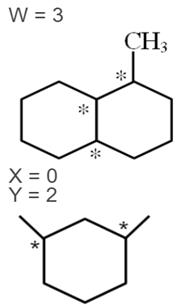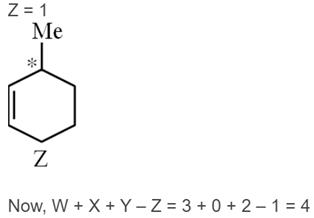Courses

# Chemistry Test 8 - Solid States, Solutions, Coordination Compounds, Alcohol Phenol And Ether, Polymers

## 25 Questions MCQ Test Mock Test Series for JEE Main & Advanced 2022 | Chemistry Test 8 - Solid States, Solutions, Coordination Compounds, Alcohol Phenol And Ether, Polymers

Description
This mock test of Chemistry Test 8 - Solid States, Solutions, Coordination Compounds, Alcohol Phenol And Ether, Polymers for JEE helps you for every JEE entrance exam. This contains 25 Multiple Choice Questions for JEE Chemistry Test 8 - Solid States, Solutions, Coordination Compounds, Alcohol Phenol And Ether, Polymers (mcq) to study with solutions a complete question bank. The solved questions answers in this Chemistry Test 8 - Solid States, Solutions, Coordination Compounds, Alcohol Phenol And Ether, Polymers quiz give you a good mix of easy questions and tough questions. JEE students definitely take this Chemistry Test 8 - Solid States, Solutions, Coordination Compounds, Alcohol Phenol And Ether, Polymers exercise for a better result in the exam. You can find other Chemistry Test 8 - Solid States, Solutions, Coordination Compounds, Alcohol Phenol And Ether, Polymers extra questions, long questions & short questions for JEE on EduRev as well by searching above.
QUESTION: 1

### The molal depression constant for water is 1.86. The depression constant for 100g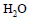is

Solution: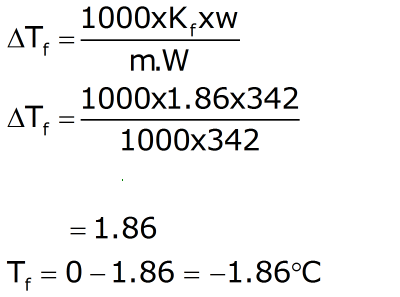QUESTION: 2

Solution:
QUESTION: 3

### The moleucular weight of NaCl (degree of dissociation=x) determined by the osmotic pressure method, is found to be different from its actual molecular wieght (M).Which of the following relationship is correct ?

Solution:
QUESTION: 4
The osmotic pressure of glucose solution (400mm),on dilution, decreased to 100 mm. The extent of dilution is
Solution:
QUESTION: 5
At what temperature does an aqueous solution containing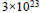molecules of a noneelectrolyte substance in 250g of water freeze ?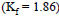Solution:
QUESTION: 6
The vapour pressure of a solvent decreased by 10mmHg when a nonvolatile solute was added to the solvent. The mole fraction of the solute in the solution
is 0.2. What would be the mole fraction of the solvent if the decrease in the vapour pressure is to be 20 mmHg
Solution:
The equation governing the change in the vapor pressure when you use a nonvolatile liquid or solid is just a special case of Raoult's Law, Ptot = PaXa + PbXb. Since the second component has a negligible vapor pressure (nonvolatile), Raoult's law reduces to

P = P0X
where P is the vapor pressure of the mixture, P0 is the vapor pressure of the pure solvent and X is the mole fraction of the solvent.
Mole fraction of solute = 0.2
so mole fraction of solvent = 0.8
So
P-10 = 0.8(P)
So P= 10/0.2 = 50

In second case
50-20 = x.50
30/50= x
So mole fraction of solvent = 0.6
QUESTION: 7

Ethyl chloride is converted into diethyl ether by

Solution:
QUESTION: 8

A metal crystallizes with a face-centered cubic lattice. The edge of the unit cell is 408 pm. The diameter of the metal atom is

Solution:
For an FCC cubic lattice:
r=a√2/4
where,
r is the radius of atom
a is the edge length of unit cell
r=408x1.414/4
=144.228 pm
Therefore, diameter of metal atom = 2 x 144.228 pm = 288.456 pm
QUESTION: 9
Alkene R–CH–CH =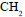reacts with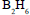in the presence of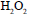to give
Solution:
QUESTION: 10
The order of reactivity of the following alcohols towards conc. HCl is
i.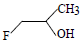ii.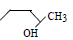iii.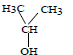iv.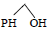Solution:
QUESTION: 11
Alcohol can be obtained by all methods except
Solution:
Bcz water
or alcohols would protonate and thus
destroy the Grignard reagent
, because the
Grignard
carbon is highly nucleophilic.

QUESTION: 12
Increasing order of acid strength among tertiary butanol,isopropanol and ehanol is
Solution:
QUESTION: 13
Chlorobenzene on fusing with solid NaOH gives
Solution:
QUESTION: 14
For the reaction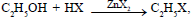the order of reactivity is
Solution:
QUESTION: 15
Benzyl alcohol on reaction with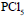gives
Solution:
QUESTION: 16

In the reaction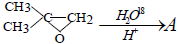the product (A) has the structure

Solution:
QUESTION: 17
Propene is allowed to react with HI. The product (A) is then treated with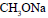to give a new product (B)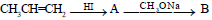The final product (B) is
Solution:
QUESTION: 18
The reaction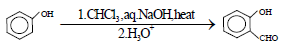is an example of
Solution:
QUESTION: 19
The utility of the polymers in various fields is due to their mechanical properties like tensile strength,elasticity, toughness etc.These properties mainly depend upon intermolecular forces like van der Waal’s forces and hydrogen bonding operating in polymer molecules. Polymers have been classified on this
basis,e.g.(1) Elastomers (2) Thermoplacstics (4) Thermosetting. Hence
The molecular forces of attraction are weakest in
Solution:
QUESTION: 20
The utility of the polymers in various fields is due to their mechanical properties like tensile strength,elasticity, toughness etc.These properties mainly depend upon intermolecular forces like van der Waal’s forces and hydrogen bonding operating in polymer molecules. Polymers have been classified on this
basis,e.g.(1) Elastomers (2) Thermoplacstics (4)Thermosetting. Hence
Which of the following have usually a linear structure
Solution:
*Answer can only contain numeric values
QUESTION: 21

Given length of side of HCP is 100/√2 pm. The volume  of HCP unit cell is (in pm3) x × 10then x will be–

Solution: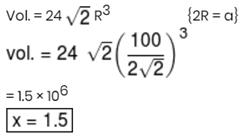*Answer can only contain numeric values
QUESTION: 22

The radius of A+ in ionic compound AB is .225Å If AB has ZnS structure, the ideal radius of B will be (in PM)

Solution: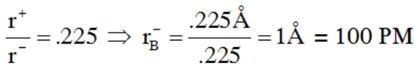*Answer can only contain numeric values
QUESTION: 23

K[Co(CO)4], K­3[Co(CN)6] Find the difference between oxidation number of central metal in the given complexes.

Solution:
*Answer can only contain numeric values
QUESTION: 24

Total no. of unpaired electrons in [Fe(H2O)6]2+ are?

Solution:
*Answer can only contain numeric values
QUESTION: 25

Number of chiral carbon atoms in the given compounds are W, X, Y and Z respectively then Calculate the I, II, III & IV value of W + X + Y– Z.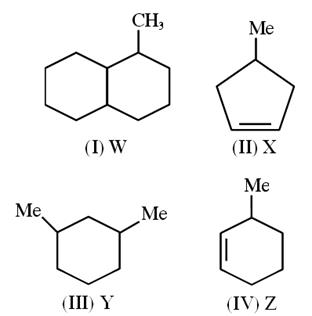Solution: